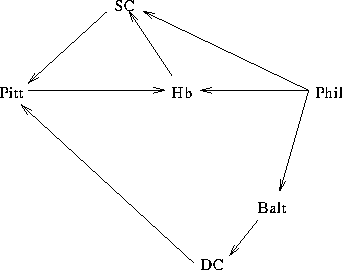Tom Kelliher, CS23

Mar. 31, 1997

• Reading: Carrano, Chapters 6 and 7.

• Next programming assignment: Project 15, pg. 350:
1. Handout on Wednesday.

2. Discussion on Friday.

3. Preview of needed classes: sorted linked list, queue.

4. Where to find Carrano's code.

ADT stack: a fundamental data structure.

• Expression evaluation.
• Activation records.

1. CreateStack() --- Create an empty stack.
2. DestroyStack()
3. StackIsEmpty()
4. Push(NewItem, Success) --- Adds NewItem to the top of the stack.
5. Pop(Success) --- Removes the most recently pushed item from the stack.
6. GetStackTop(StackTop, Success) --- Retrieves the value of the most recently pushed item.

# A Simple Example

Consider evaluating postfix expressions:

```23 17 + 18 +
2 5 + 4 2 + *
1 7 4 * + 5 +
```

An algorithm:

```CreateStack()
while not end of line
token = next token from input stream
if token is a number
Push(token, success);
else if token is an operator
GetStackTop(operand1, success)
Pop(operand1, success)
GetStackTop(operand2, success)
Pop(operand2, success)
result = the value of the operator in token applied to
operand1 and operand2
Push(result, success)
else   // invalid token
terminate
end_if
end_while

DestroyStack()
```

Where should errors be checked?

# A Sample Implementation

stack.h:

```#ifndef __STACK_H
#define __STACK_H

#include <stdlib.h>

struct stackItem
{
stackItem* next;
int value;
};

typedef stackItem* stackPtr;

class Stack
{
private:
stackPtr s;

public:
Stack() : s(NULL) {}
~Stack();
Stack(const Stack& oldStack);   // copy constructor
int StackIsEmpty(void);
void Push(int newItem, int& success);
void Pop(int& success);
void GetStackTop(int& stackTop, int& success);
};

#endif
```

stack.cc:

```#include "stack.h"
#include <stdlib.h>
#include <assert.h>

Stack::~Stack()
{
stackPtr tmp;

while (s != NULL)
{
tmp = s;
s = s->next;
delete tmp;
tmp = NULL;
}
}

Stack::Stack(const Stack& oldStack)
{
stackPtr oldPtr = oldStack.s;
stackPtr ptr;

if (oldStack.s == NULL)
{
s = NULL;
return;
}
else
{
s = new stackItem;
assert (s != NULL);
ptr = s;
ptr->next = NULL;
ptr->value = oldPtr->value;
oldPtr = oldPtr->next;
}

while (oldPtr != NULL)
{
ptr->next = new stackItem;
assert (ptr->next != NULL);
ptr = ptr->next;
ptr->next = NULL;
ptr->value = oldPtr->value;
oldPtr = oldPtr->next;
}
}

int Stack::StackIsEmpty(void)
{
return s == NULL;
}

void Stack::Push(int newItem, int& success)
{
stackPtr tmp;

tmp = new stackItem;

if (!(success = tmp != NULL))
return;

tmp->value = newItem;
tmp->next = s;
s = tmp;
}

void Stack::Pop(int& success)
{
stackPtr tmp;

if (s == NULL)
success = 0;
else
{
success = 1;
tmp = s;
s = s->next;
delete tmp;
}
}

void Stack::GetStackTop(int& stackTop, int& success)
{
if (s == NULL)
success = 0;
else
{
success = 1;
stackTop = s->value;
}
}
```

Example usage:

```#include "stack.h"
#include <iostream.h>

int main()
{
Stack s;
int tmp, success;

s.Push(2, success);   // build s
s.Push(5, success);

Stack t = s;   // call copy constructor to make a copy
// different from regular assignment

cout << "s:\n";

s.GetStackTop(tmp, success);
cout << tmp << endl;

s.Pop(success);
s.GetStackTop(tmp, success);
cout << tmp << endl;
s.Pop(success);
cout << s.StackIsEmpty() << endl;

cout << "t:\n";

t.GetStackTop(tmp, success);
cout << tmp << endl;

t.Pop(success);
t.GetStackTop(tmp, success);
cout << tmp << endl;
t.Pop(success);
cout << t.StackIsEmpty() << endl;

return 0;
}
```

# Backtracking Example: Searching a Graph

Problem: Finding a set of airline flights to travel from one city to another, if one exists.

Consider a flight map:How is it represented?

City file (graph vertices):

```Phil
Hb
SC
Pitt
Balt
DC
```

Flight file (graph edges):

```Phil SC
Phil Hb
Phil Balt
SC Pitt
Hb SC
Pitt Hb
Balt DC
DC Pitt
```

Request file:

```Phil DC
DC Phil
```

## Basic Data Structures

Implemented as various classes.

1. Adjacency lists to represent flight map.
2. Vector of unvisited cities.
3. Itinerary stack.
4. Functions to map from a city name to a city number and vice-versa.

## Algorithm

```read city file, construct names array, visited array,

open request file;

while there is a request
{
check its validity;
mark departure point visited and push onto stack;

while the stack is not empty and the destination
is not on top of stack
{
if there are no unvisited cities on the adjacency list for
the top of stack city
pop it from the stack;
else
mark that city as visited and push onto stack;
}

if the stack is empty
no flight path exists
else
print the flight path
```

# Stacks and Recursion

What does recursion use?

What data structure is used for activation records?

Thomas P. Kelliher
Sun Mar 30 17:55:01 EST 1997
Tom Kelliher